Mathematical and Physical Journal
for High Schools
Issued by the MATFUND Foundation
 Already signed up? New to KöMaL?

# KöMaL Problems in Mathematics, September 2006

Show/hide problems of signs:## Problems with sign 'K'

Deadline expired on October 10, 2006.

K. 85. Annie and Bennie were playing with a chair. When Annie was standing on the chair and Bennie on the floor, the top of Annie's head was 30 cm higher than Bennie's. When they exchanged places, Bennie standing on the chair and Annie on the floor, the top of Bennie's head was half a metre higher than Annie's. How tall is the chair?

(6 pont)

solution (in Hungarian), statistics

K. 86. A large cube is put together out of small unit cubes. There are 80 small cubes altogether that lie along the edges or at the corners of the large one. How many small cubes does the large cube consist of?

(6 pont)

solution (in Hungarian), statistics

K. 87. The growth of an isosceles Pythagoras tree has the following pattern: In the first year, the tree grows its trunk, which is a square. In the second year, an isosceles right-angled triangle grows on top, such that its hypotenuse is the top side of the square, and then the first two branches, also square in shape, grow from the legs of the triangle. Then this pattern repeats every year, that is, an isosceles right triangle grows on the top of each branch and its legs grow two new square branches. Given that the trunk (i.e. the first square) is 8 metres wide, calculate the height and the width of the tree at the end of the fourth year. (Hint: Draw a diagram of the tree to scale on squared paper. Let one little square represent 2 metres.)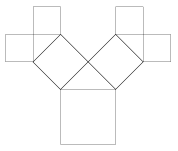(6 pont)

solution (in Hungarian), statistics

K. 88. There are four ninth-grade classes at a school. The four classes went on a four-day camp. Each class went hiking on one of the four days, while the other classes took part in presentations and other activities. On Monday, class A went hiking and thus 81 students remained in the camp. On Tuesday, class B were hiking and thus 79 students remained in the camp. On Wednesday, class C were hiking and 75 students stayed in the camp. Finally, on Thursday, with class D hiking, 80 students stayed in the camp. How many students are there in each class?

(6 pont)

solution (in Hungarian), statistics

K. 89. Find the radius of the three congruent circles in the Figure, given that the side of the equilateral triangle is 12 cm.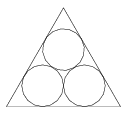(6 pont)

solution (in Hungarian), statistics

K. 90. The first nine positive integers can be written in the 9 fields bounded by the rings in the logo of the Olympic Games so that the sum of the numbers in each ring is 14. Such an arrangement is shown in the Figure. How can the numbers be arranged so that the sum is 13 in each ring? Find as many solutions as you can.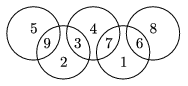(6 pont)

solution (in Hungarian), statistics## Problems with sign 'C'

Deadline expired on October 16, 2006.

C. 860. A survey carried out on a sample of 500 revealed that 46% of those asked liked strawberry ice cream, 71% liked vanilla and 85% liked chocolate ice cream. Is there anyone in the sample who likes all three flavours?

(5 pont)

solution (in Hungarian), statistics

C. 861. In a partial Solar Eclipse, the apparent diameters of the Sun and the Moon were equal. In the time instant when the eclipse was at its maximum, the edge of the Moon's shadow passed through the centre of the Sun's face. What percentage of the Sun's face was eclipsed?

(5 pont)

solution (in Hungarian), statistics

C. 862. Find the number pairs x, y for which the following inequality is true: 2|x+y||x|+|y|.

(5 pont)

solution (in Hungarian), statistics

C. 863. Find the integer solutions x, y of the equation x6-y2=648.

(5 pont)

solution (in Hungarian), statistics

C. 864. A triangle is drawn on squared paper. The lengths of the sides are,and 5 units. Prove that the measure of the smallest angle is 45o.

(Suggested by G. Holló, Budapest)

(5 pont)

solution (in Hungarian), statistics## Problems with sign 'B'

Deadline expired on October 16, 2006.

B. 3922. I have a six-digit number in mind. By transferring the first digit to the end, I would obtain three times the original number. What is my number?

(3 pont)

solution (in Hungarian), statistics

B. 3923. A diagonal is drawn in a few fields of a chessboard, such that no two of them have a common point. What is the maximum possible number of diagonals that can be drawn in this way?

(Based on a problem of the Zrínyi Competition)

(4 pont)

solution (in Hungarian), statistics

B. 3924. 27 regular dice are glued together to form a 3×3×3 large cube. On every pair of faces of the dice glued together, there are the same number of spots. How many spots are there on the surface of the large cube?

(3 pont)

solution (in Hungarian), statistics

B. 3925. Let T denote the orthogonal projection of the midpoint F of the circular arc ABC onto the broken line ABC. Prove that T halves the length of the broken line.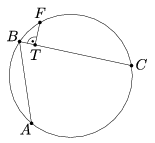(4 pont)

solution (in Hungarian), statistics

B. 3926. Ann and Bob are playing the following game: There are 10 heaps of pebbles in front of them. The first heap contains 1 pebble, the second one contains 2, the third one contains 3, and so on, the tenth heap contains 10 pebbles. They take turns in carrying out one of the following two moves: they either divide a heap into two smaller ones or remove one pebble from one heap. A player who cannot do so any more will lose the game. Determine which player has a winning strategy, given that Ann starts the game.

(5 pont)

solution (in Hungarian), statistics

B. 3927. Vertex A of a tetrahedron ABCD is reflected about B, B is reflected about C, C about D and D about A. Let the respective reflections be A', B', C' and D'. By what factor is the volume of the tetrahedron A'B'C'D' larger than that of the original tetrahedron ABCD?

(Suggested by G. Holló, Budapest)

(4 pont)

solution (in Hungarian), statistics

B. 3928. P is an interior point of a circle of radius r. Let the arms of a right angle with vertex P intersect the circle at A and B. Complete the triangle BPA to form a rectangle PAQB. What curve will the point Q describe while the right angle turns about P?

(4 pont)

solution (in Hungarian), statistics

B. 3929. Let {r} denote the number obtained by taking away from r the greatest integer not greater than r. Prove that the equation {x3}+{y3}={z3} has infinitely many solutions, such that x, y and z are rational numbers, but none of them are integers.

(5 pont)

solution (in Hungarian), statistics

B. 3930. Prove that by drawing some noncrossing diagonals it can be divided into triangles such that each vertex of the polygon belongs to an odd number of them.

(4 pont)

solution (in Hungarian), statistics

B. 3931. Find a polynomial p of rational coefficients, such that.

(Based on the suggestion by Ervin Fried, Budapest)

(5 pont)

solution (in Hungarian), statistics## Problems with sign 'A'

Deadline expired on October 16, 2006.

A. 404. The vertices of a regular 2n-gon are. Call a diagonal ViVj even if i and j have the same parity. Dissect the polygon into triangles arbitrarily drawing 2n-3 nonintersecting diagonals. The following operation is allowed on this dissection: Choose two vertices, Vi and Vj, which are either consecutive or they are connected by a diagonal used for the dissection. Then, on one side of the line ViVj replace each diagonal by its mirror image through the perpendicular bisector of ViVj (see the figure). Prove that, starting from an arbitrary dissection and applying this operation several times, it can be achieved that all even diagonals used for the dissection connect only vertices of even indices.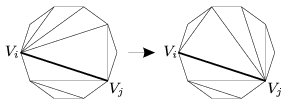(Based on the sixth problem of the 47th IMO, Slovenia)

(5 pont)

statistics

A. 405. The real numbers a, b, c, x, y, z satisfy abc>0 and xyz>0. Prove that(Korean competition problem)

(5 pont)

solution, statistics

A. 406. Assign to each side b of a convex polygon P the maximum area of a triangle that has b as a side and is contained in P. Show that the sum of the areas assigned to the sides of P is at most three times the area of P.

(Based on the second problem of the 47th IMO, Slovenia)

(5 pont)

statistics